# JEE Main 2020 Question Paper with Solution (Jan 9th Second shift)

## 9th January, Afternoon Session ( 2:30 pm to 5:30 pm)

JEE Mains 2020 January session is almost over now, the JEE main 2020 exam was conducted from January 7th to January 9th in 6 shifts. The last paper of JEE Main was conducted today January 9 in two shifts. First shift timings were 9:30 am to 12:30 pm and second shift timings were 2:30 pm to 5:30 pm. In this article, you will get JEE main 2020 question paper with solution (Jan 9th Second shift). As we all know that NTA is conducting JEE main 2020 exam, this time paper has been more balanced and providing an equal chance to all aspirants. The JEE mains 2020 Jan 9 second shift exam solutions are created by experts on the basis of memory-based JEE mains question available from aspirants. Stay tuned with us for more updates and solutions.

Exam date and Shift Article URL

JEE Main 2020 exam - Chemistry ( 9th January Second shift)

Q1) In which compound C–Cl bond length is shortest?

(1) Cl–CH=CH2

(2) Cl–CH=CH–CH3

(3) Cl–CH=CH–OCH3

(4) Cl–CH=CH–NO2

Solution

Resonance form of Cl–CH=CH–NO2 is more stable than resonance form of any other given compounds. Hence, double bond character in carbon-chlorine bond is maximum and bond length is shortest.

Option 4 is correct.

Q2) Amongst the following which has minimum conductivity.

(1) Distilled water

(2) Sea water

(3) Saline water used for intra venous injection

(4) Well-water

Solution

Distilled water is de-ionised water.

option (1) is correct.

Q3) Number of sp2 hybrid orbitals in a molecule of benzene.

1. 6

2. 12

3. 18

4. 24

Solution:  18 sp2 hybrid orbitals are present in a molecule of benzene.

Therefore,  the option number (3) is correct.

Q4) Biochemical oxygen demand (BOD) is defined as _______ in ppm of O2.
(1) Required to sustain life
(2) The amount of oxygen required by bacteria to break down the organic matter present in a certain volume of a sample of water.
(3) The amount of oxygen required by anaerobic bacteria to break down the inorganic matter present in a certain volume of a sample of water.
(4) Required photochemical reaction to degrade waste

Solution

The amount of oxygen required by bacteria to break down the organic matter present in a certain volume of a sample of water, is called Biochemical Oxygen Demand (BOD).

Option 2 is correct.

Q5) Which of the following reaction will not form racemic mixture as product?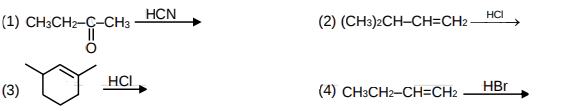Solution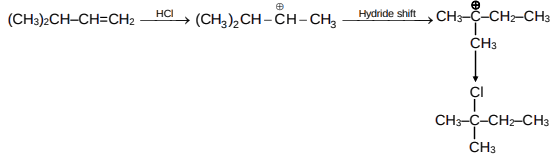Opton  2 is correct.

Q6)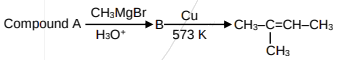Percentage carbon in compound A is:

Solution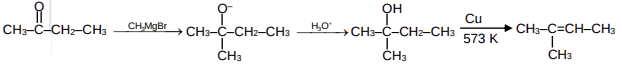Compounds is: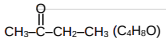Q7) 0.1 ml of an ideal gas has volume 1 dm3 in a locked box with frictionless piston. The gas is in thermal equilibrium with excess of 0.5 m aqueous ethylene glycol at its freezing point. If the piston is released all of a sudden at 1 atm then determine the final volume of gas in dm3 (R = 0.08 atm L mol–1 K–1 Kf = 2.0 K molal–1).

Solution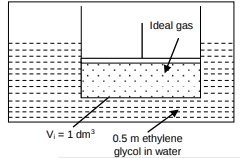After releasing piston P1V1 = P2V2
2.176 x 1 = 1 x V2
V2 = 2.176 dm3

Q8) One litre sea water (d = 1.03g/cm3) contains 10.3 mg O2 gas. Determine concentration of O2 in ppm.

Solution

Thus, the correct answer is 10.

Q9) Lacto bacillus has generation time 60 min. at 300 K and 40 min. at 400 K. Determine activation energy in mol kJ/mol. (R = 8.3 J K–1mol–1) [ln(2/3) = -0.4].

Solution

ln(3/2) × 8.3 × 1200 = Ea
Ea = 0.4 × 8.3 × 1200
Ea = 3984 J/mol.
Ea = 3.984 kJ/mol

Thus, the correct answer is 3.98

Q10) Total number of Cr–O bonds in Chromate ion and dichromate ion is:

Solution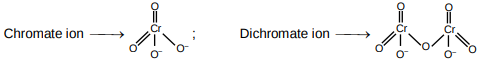Total number of Cr and O bonds is 12.

Q11)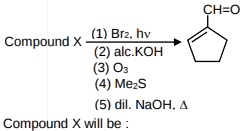SolutionQ12)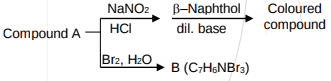Compound A will be: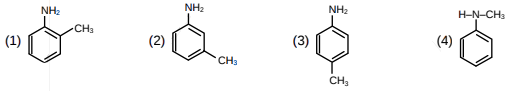Solution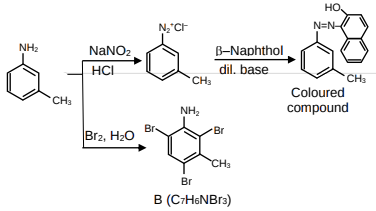Q13) The order of basic character is :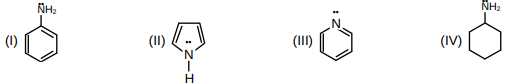(1) I > II > III > IV
(2) IV > III > I > II
(3) II > I > III > IV
(4) IV > I > II > III

Solution
Basic strength depends upon availability of lone pairs. Greater the resonance of lone pairs lesser the basic strength.

Option 2 is correct.

Q14)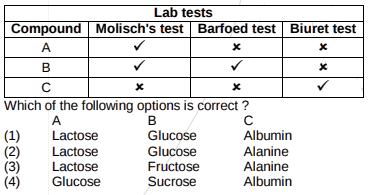Solution

 Laboratory Test Molisch's test Barfoed test Biuret test Yes Lactose, Glucose, Fructose Glucose Albumin No Sucrose Alanine

Therefore, Option(1) is correct.

Q15) 1. 5 g of Zn reacts with
(I) Excess of NaOH (II) Dilute HCl, then volume ratio of H2 gas evolved in (I) and (II) is
(1) 2 : 1

(2) 1 : 2

(3) 1 : 1

(4) 3 : 1

Solution

According to stoichiometry in both the reactions, equal number of moles of H2 are evolved.

Option 3 is correct.

Q16) Given Ksp for Cr(OH)3 is 6 × 10–31 then determine [OH].
(Neglect the contribution of OH– ions from H2O)

Solution

s           3s

Option 1 is correct.

Q17) Select the correct statements among the followings
(A) LiCl does not dissolve in pyridine
(B) Li does not react ethyne to form ethynide.
(C) Li and Mg react slowly with water.
(D) Among alkali metals Li has highest hydration tendency.

Solution

Concept Based

Option  1 is correct.

Q18) Given an element having following ionisation enthalpies IE1 = 496 kJ/mol and IE2 = 4562 kJ/mol one mole hydroxide of this element is treated separated with HCl and H2SO4 respectively. Moles of HCl and H2SO4 reacted respectively is

(1) 1, 0.5

(2) 0.5, 1

(3) 2, 0.5

(4) 0.5, 2

Solution

According to the given data of I.E, This element must belong to group 1 and thus is monovalent & form
hydroxide of the type M(OH).

Q19) Reactant A represented by square is in equilibrium with product B represented by circles. Then value of
equilibrium constant is(1) 1

(2) 2

(3) 3

(4) 4

Solution

Option 2 is correct.

Q20) Given following complexes

(I) Na4[Fe(CN)6]               (II) [Cr(H2O)6] Cl2

(III) (NEt4)2 [CoCl4]          (IV) Na3[Fe(C2O4)3]

Correct order of spin only magnetic moment for the above complexes is.

(1) (II) > (III) > (IV) > I

(2) (II) > (IV) > (III) > (I)

(3) (I) > (IV) > (III) > (II)

(4) (II) > (I) > (IV) > (III)

Solution

Option 1 is correct.

Q21) Select the correct option :
(1) Entropy is function of temperature and also entropy change is function of temperature.
(2) Entropy is a function of temperature & entropy change is not a function of temperature.
(3) Entropy is not a function of temperature & entropy change is a function of temperature.
(4) Both entropy & entropy change are not a function of temperature.

Solution

Option 1 is correct.

Q22) A compound (A ; B3N3H3Cl3) reacts with LiBH4 to form inorganic benzene (B). (A) reacts with (C) to form B3N3H3(CH3)3. (B) and (C) are respectively.
(1) Boron nitride, MeMgBr

(2) Boron nitride, MeBr

(3) Borazine, MeBr

(4) Borazine, MeMgBr

Solution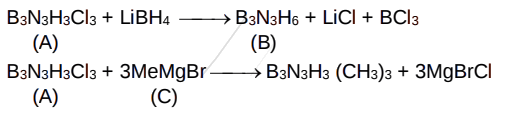Option 4 is correct.

Q23) In a box a mixture containing H2, O2 and CO along with charcoal is present then variation of pressure with the time will be as follows :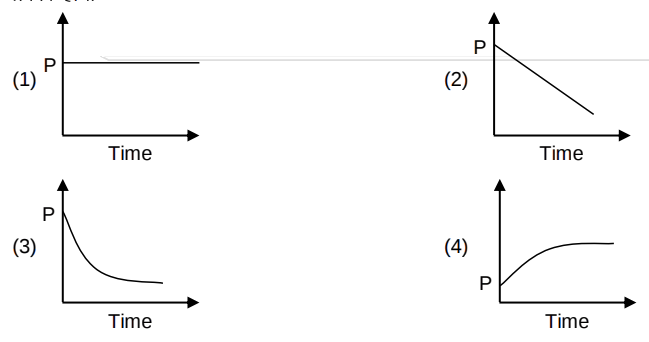Solution

Concept based

Option  3 is correct

Q24) Given complex [Co(NH3)4Cl2]. In it if Cl - Co-Cl bond angle is 90º then it is :
(1) Cis-isomer

(2) Trans- isomers

(3) Meridional and trans

(4) Cis and trans both

Solution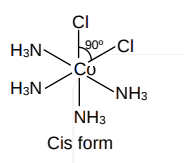Option 1 is correct.

Q25) Monomer(s) of which of the given polymer is chiral?

(1) Buna-S

(2) Neoprene

(3) Nylon-6,6

(4) PHBV

Solution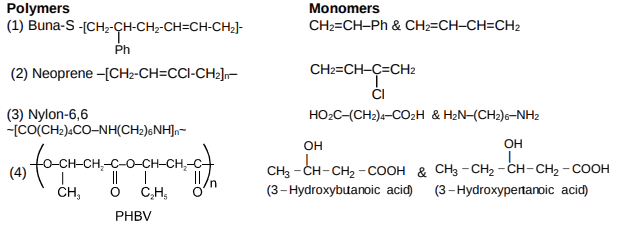In PHBV, both monomers have chiral centre.

Option 4 is correct.

JEE Main 2020 exam - Mathematics ( 9th January Second shift)

Q1. AB is focal chord at parabola , where then equation ale tangent at B is

Solution:

Q2. IF  and

Solution:

Use

Q3. If the statement  is false then p and q respectively is ?

Solution:

Q4. If ,  y(1) = 1 and y(x) = e, then x is

Solution:

Q5. If the minimum value of term free from x for is L and L2 in. Find .

Solution:

Q6. Number of common terms in both sequence 3, 7, 11, ………….407 and 2, 9, 16, ……..905 is

Solution:

Q7. Let a angle between equal to If is perpendicular to then find the value of .

Solution:

Q8. Let circles (x – 0)2 + (y – 4)2 = k and (x – 3)2 + (y – 0)2 = 12 touches each other than find the maximum value of 'k'

Solution:

Two circles touch each other if

Distance between C2(3, 0) and C1(0, 4) is either

Also

Maximum value of K is 36

Q9.

Solution:

Q10. Let the distance between plane passing through lines  and  and plane 23x – 10y – 2z + 48 = 0 is  then k is equal to

Solution:

and

Lines must be intersecting

Hence points are (-3,-2,1)

The distance of plane contains given lines from given plane is same as distance between point (–3, –2,1) from given plane.

Required distance equal to

Q11.   then find the area bounded by f(x) and g(x) from

Solution: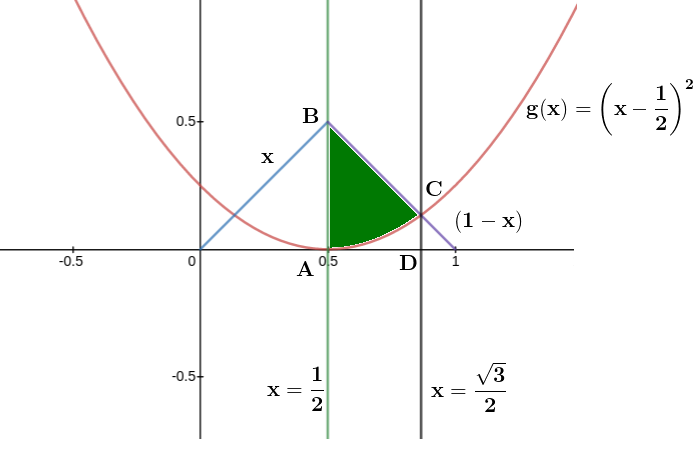Required area = Area of trapezium ABCD -

Q12. z is a complex number such that |Re(z)| + |Im (z)| = 4 then |z| can't be

Solution:

z = x + iy

|x| + |y| = 4

Minimum value of |z| =

Maximum value of |z| = 4

So |z| can't be

Q13. If f(x) =  and a – 2b + c = 1, than

(1) f(50) = 1

(2) f(–50) = – 1

(3) f(50) = 501

(4) f(50) = – 501

Solution:

Q14. Let an is a positive term of a GP and

Solution:

From above, r = 2

Q15. Let probability distribution is

then value of p(x > 2) is

Solution:

Q16. Let and then

Q17. Let both root of equation ax2 – 2bx + 5 = 0 are and root of equation x2 – 2bx – 10 = 0 are and . Find the value of

Solution:

ax2 – 2bx + 5 = 0 having equal roots or  and

Put  in the second equation

Q18.  then correct choice is

(1) F(x) has local minimum at x = 1

(2) F(x) has local maximum at x = 1

(3) F(x) has point of inflection at x = 1

(4) F(x) has no critical point

Solution:

Q19. Let and find at

Solution:

Q20. If 7x + 6y – 2z = 0; 3x + 4y + 2z = 0 and x – 2y – 6z = 0 then which option is correct

(1) no. solution

(2) only trivial solution

(3) Infinite non trivial solution for x = 2z

(4) Infinite non trivial solution for y = 2z

Solution:

so infinite non-trivial solution exist

Q21.  then ordered pair   is

Solution:

Q22. If  = A then the value of x at which  is discontinuous (where [.] denotes greatest integer function)

Solution:

Q23. Let x + 6y = 8 is tangent to standard ellipse where the minor axis is , then eccentricity of an ellipse is

Solution:

Q24. If f(x) and g(x) are continuous functions, fog is identity function, g'(b) = 5 and g(b) = a then f'(a) is

Solution:

JEE Main 2020 exam - Physics ( 9th January Second shift)

Q-1

A mass m attached to spring of natural length  and spring constant k. One end of string is attached to centre of disc in horizontal plane which is being rotated by constant angular speed . Find extension per unit length in spring (given  )

Solution-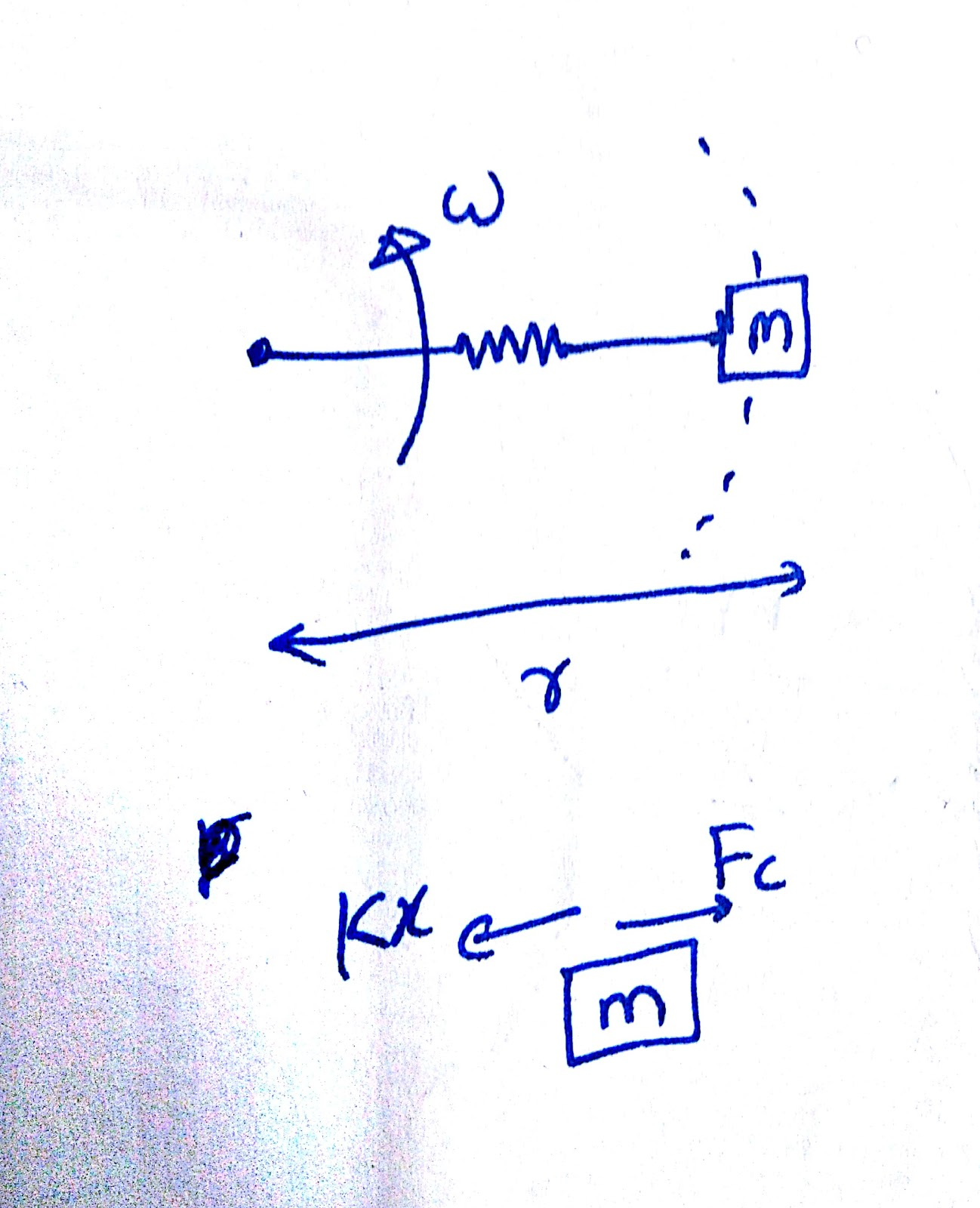As natural lentgh=l0

Let elongation=x

Mass m is moving with angular velocity  in a radius r

where

Due to elongation x spring force is given by

And

as

So

using

So

Q-2

A loop of radius R and mass m is placed in a uniform magnetic field B with its plane perpendicular to the field. Current I is flowing in it. Now loop is slightly rotated about its diameter and released. Find time period of oscillation

Solution-

Q-3

A string of mass per unit length is fixed at both ends under the tension 540 N. If the string is in resonance with consecutive frequencies 420 Hz and 490 Hz. Then find the length of the string?

(1) 2.1 m (2) 1.1 m (3) 4.8 m (4) 4.2 m

Solution-

Fundamental frequency   = 490 – 420 = 70 Hz

Q-4

Solution:-

Q-5

Solution:-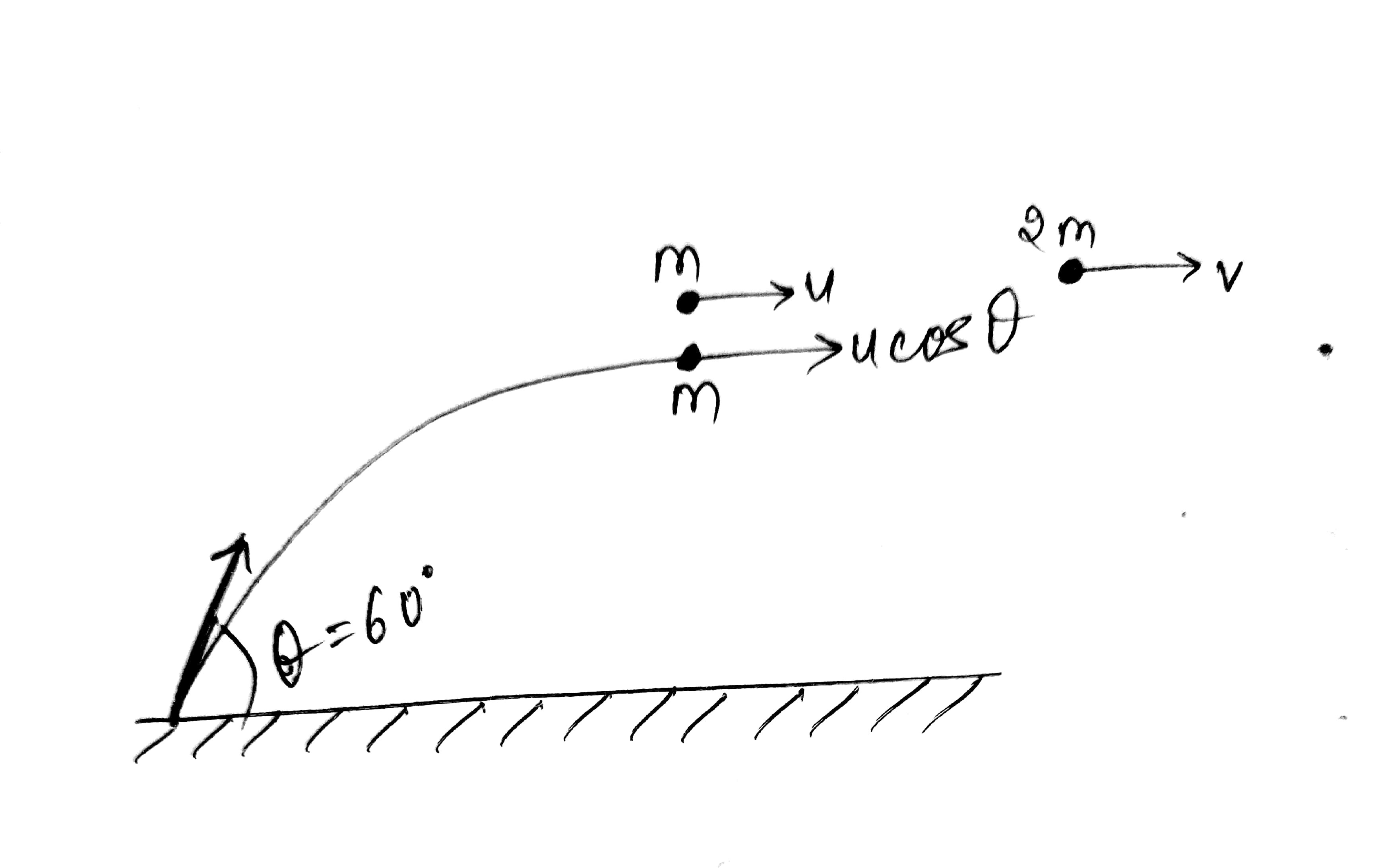Q-6

Solution:-

Q-7

Solution:-

Q-8

Solution:-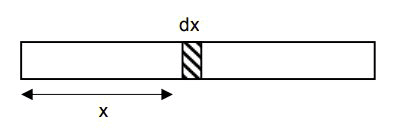Q-9

Solution:-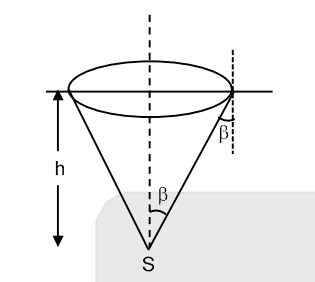Q-10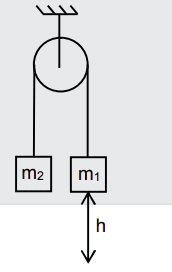Solution:-

So

Q-11

Find the current supplied by the battery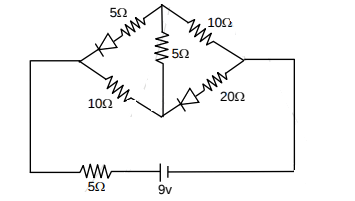(1) 0.1 A (2) 0.3 A (3) 0.4 A (4) 0.5 A

Solution-

Both diodes are in reverse biased

So new circuits can be drawn as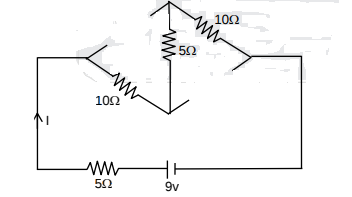So

Q-12

An AC source is connected to the LC series circuit with V = 10 sin (314t). Find the current in the circuit as a function of time? (L = 40 mH, C = 100 F)

(1) 10 sin (314t) (2) 5.2 sin (314t) (3) 0.52 sin (314t) (4) 0.52 cos (314t)

Solution-

As

But R=0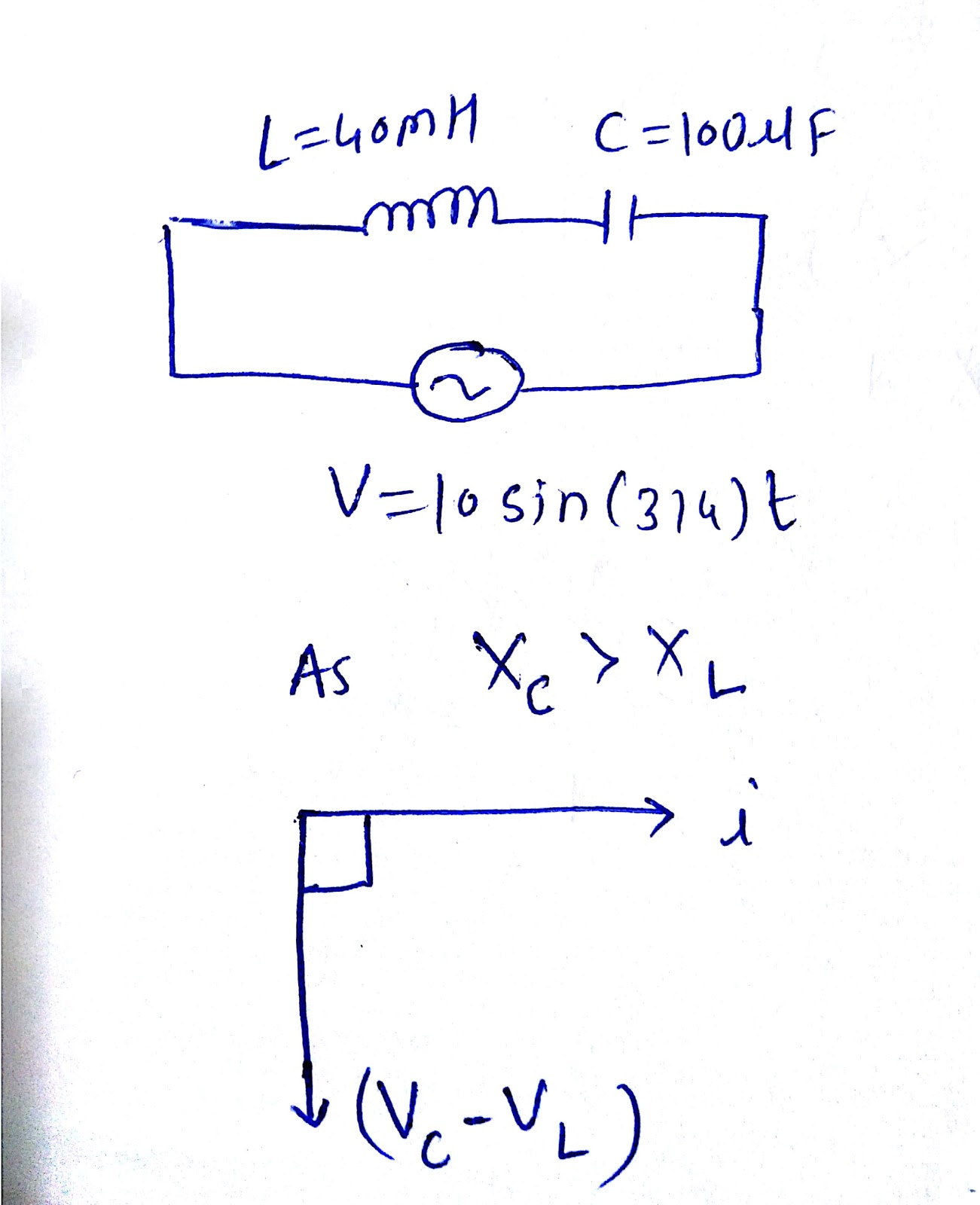Q-13

Solution:-

Q-14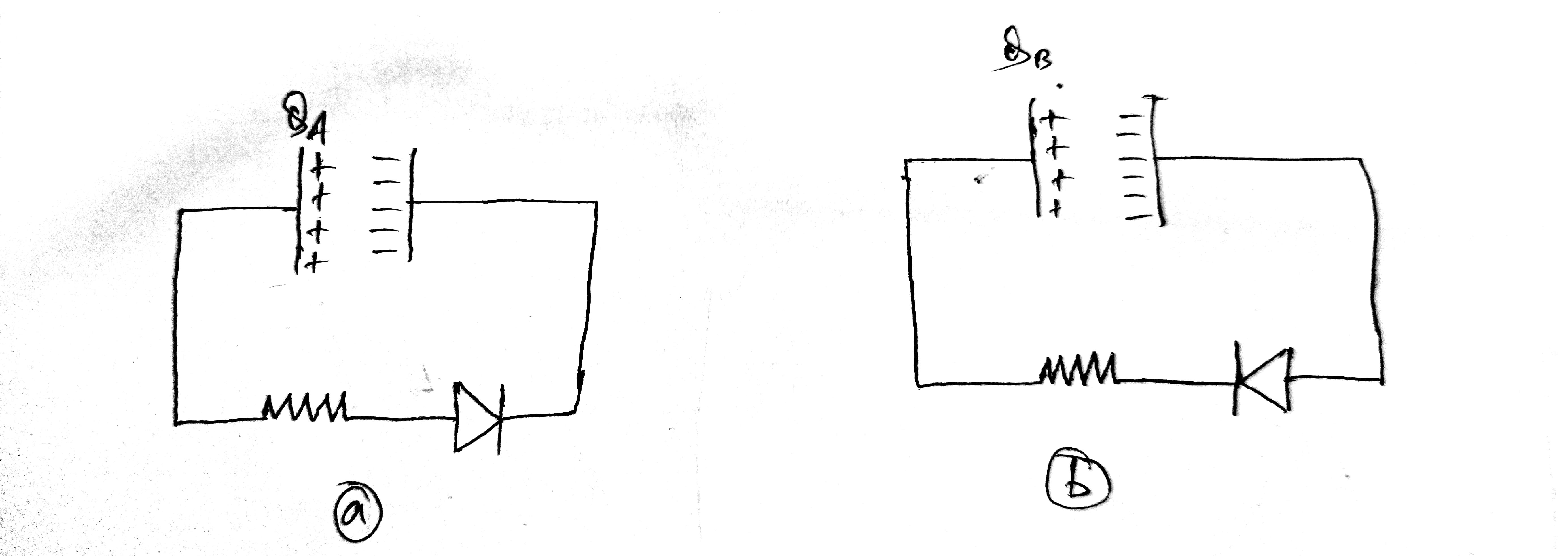Solution:-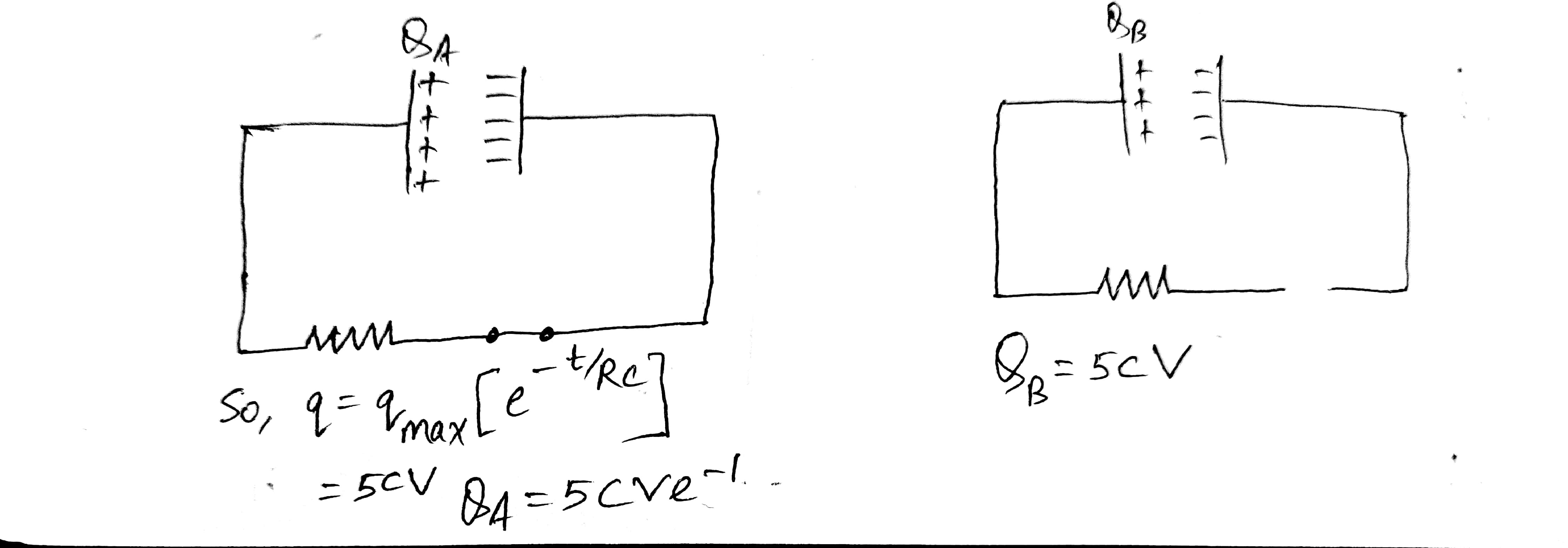Q-15

Solution:-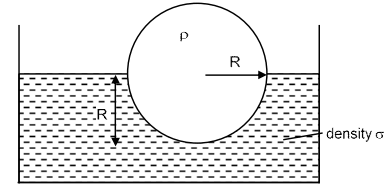Q-16

An EM wave is travelling in  direction. Axis of polarization of EM wave is found to be   . Then equation of magnetic field will be -

Solution -

EM wave is in direction -

As we know that the axis of polarisation of the Em wave is same as Electric field direction that is -

direction of propagation of EM wave =

And the equation of the electromagnetic waves will be in terms of the

So by concluding the above result we can deduce that the option (2) is correct.

Q-17

Different value of a, b and c are given and their sum is d. Arrange the value of d in increasing order -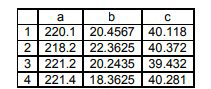Solution -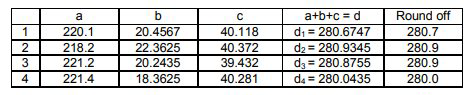So the option (3) is correct.

Q-18

Two gases Ar (40) and Xe (131) at same temperature have same number density. Their diameters are 0.07 nm and 0.10 nm respectively. Find the ratio of their mean free time

(1) 1.03                 (2) 2.04                   (3) 3.04                     (4) 2.40

Solution -

So the option (2) is correct.

Q-19

A particle start moving with velocity   from origin and acceleration   .when y co-ordinate of particle is 32 m. then x co-ordinate at that instant will be

(1) 48 (2) 60 (3) 12 (4) 24

Solution-

Q.20

An electron (-|e|, m) is released in Electric field E from rest. rate of change of de-Broglie wavelength with time will be.

Sol.

Q.21.

In YDSE pattern with light of wavelength  , 15 fringes are obtained on a certain segment of

screen. If number of fringes for light of wavelength   on same segment of screen is 10, then the value
of   is-

Solution-

.

Q.22

If in a meter bridge experiment, the balancing length  was 25 cm for the situation shown in the figure. If the length and diameter of the of wire of resistance R is made half, then find the new balancing length in centimetre is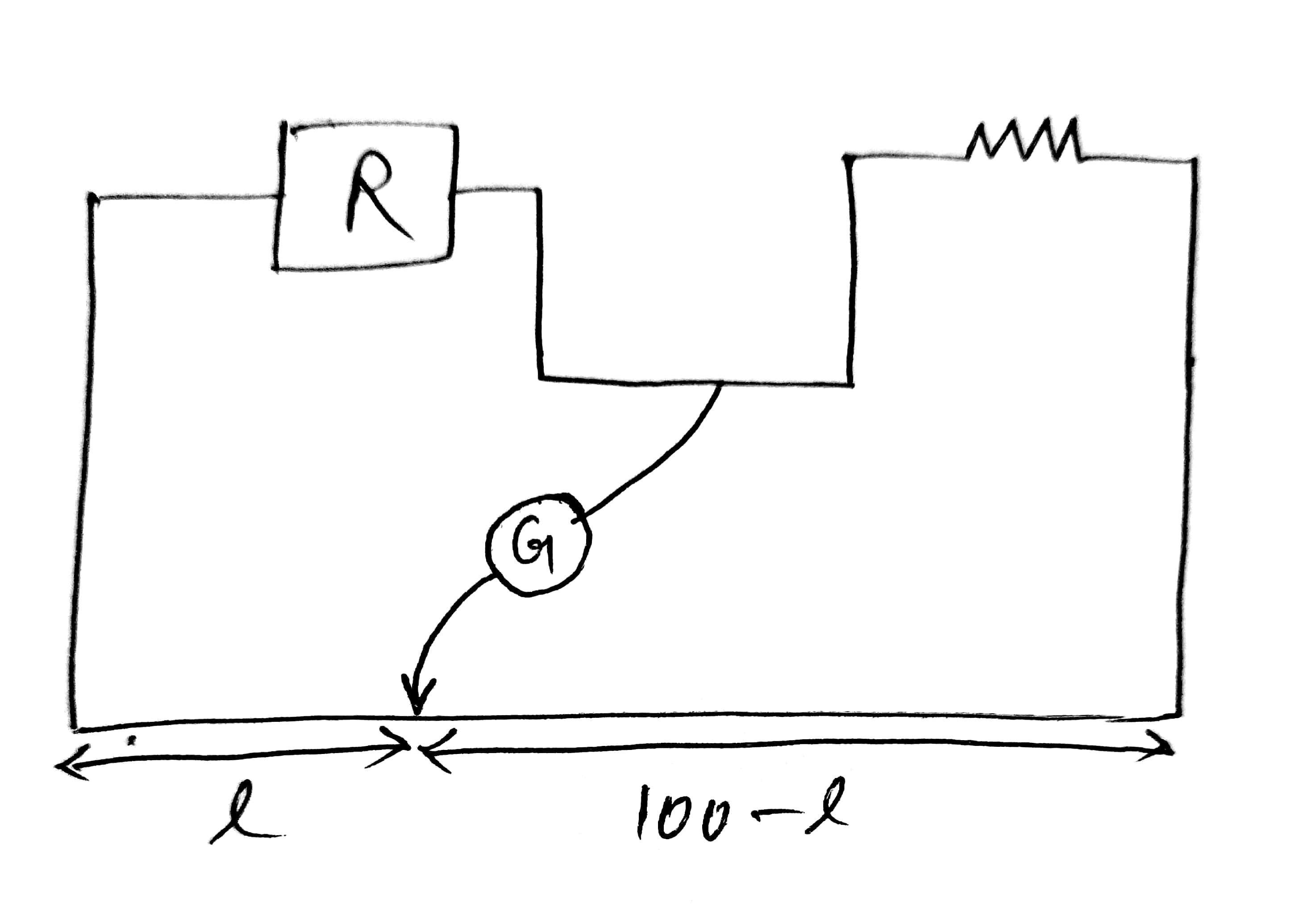Sol.

Q.23

Find the power loss in each diode (in mW), if potential drop across the zener diode is 8V.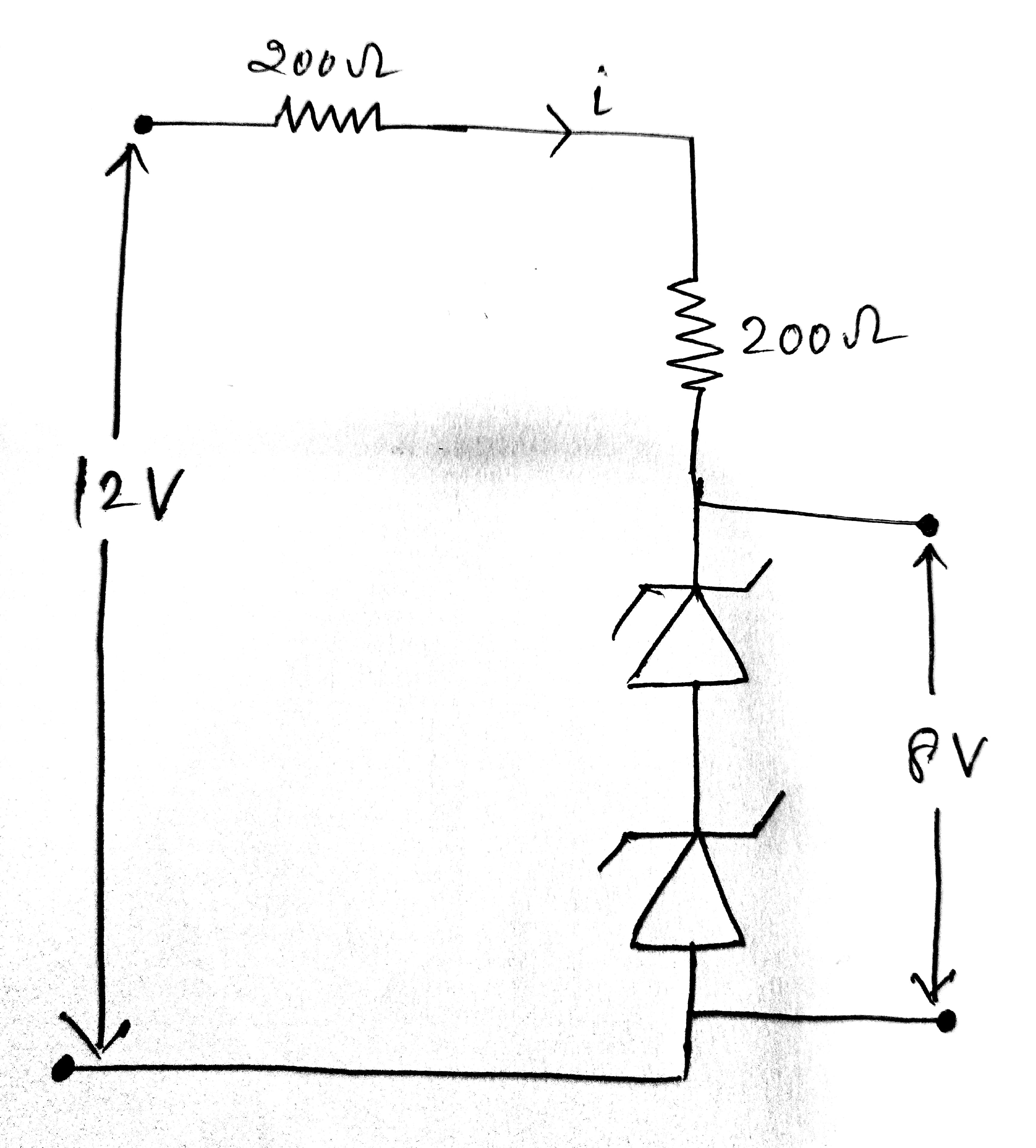Sol.

Q.24

An ideal gas at initial temperature 300 K  is compressed adiabatically  of its initial volume. The gas is then expanded isobarically to double its volume. Then final temperature of gas round to nearest integer is:

Sol.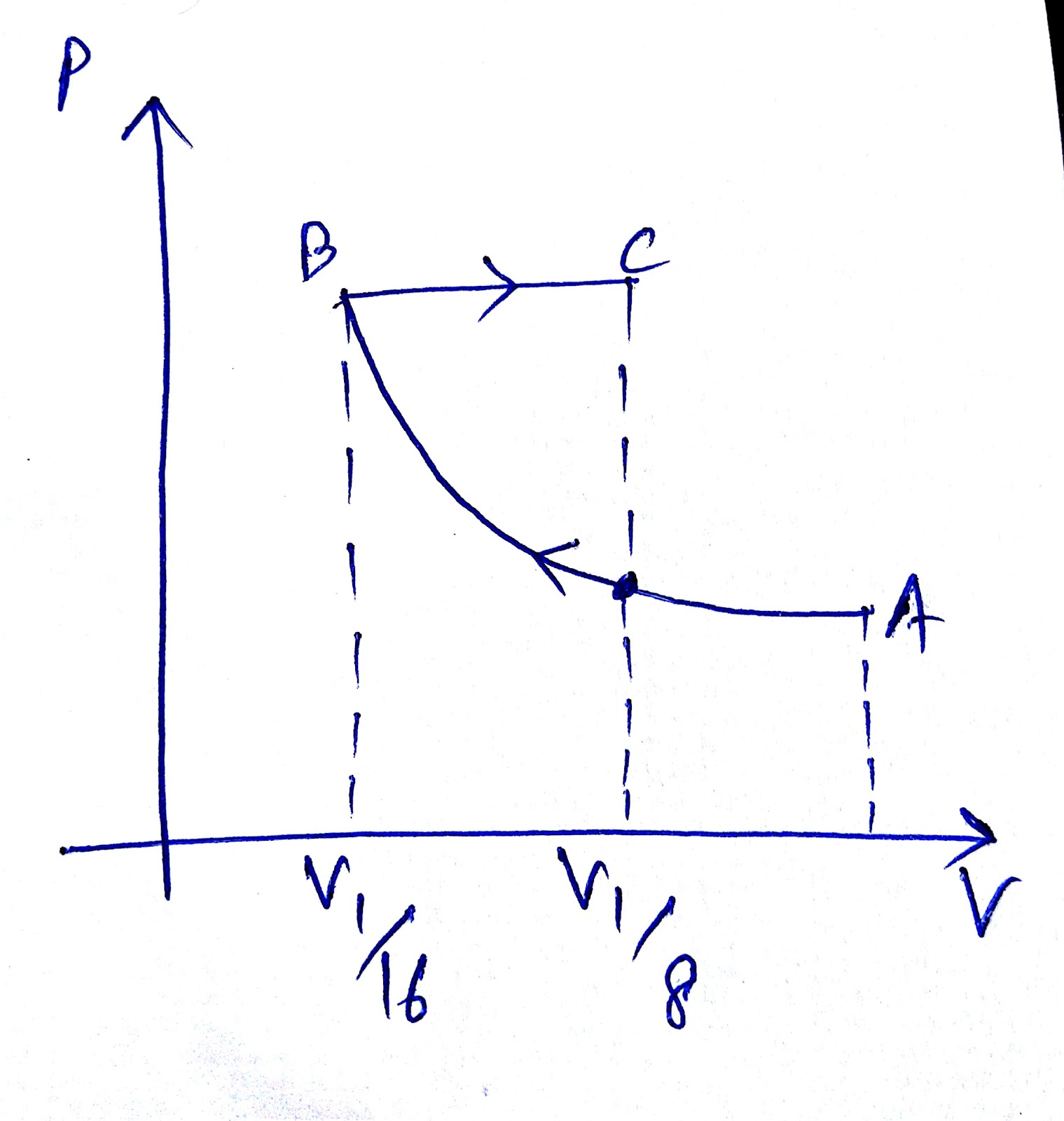= constant

= constant

Now for BC process BC

Q.25

If electric field in the space is given by  , and electric flux through ABCD is  and
electric flux through BCEF is    then find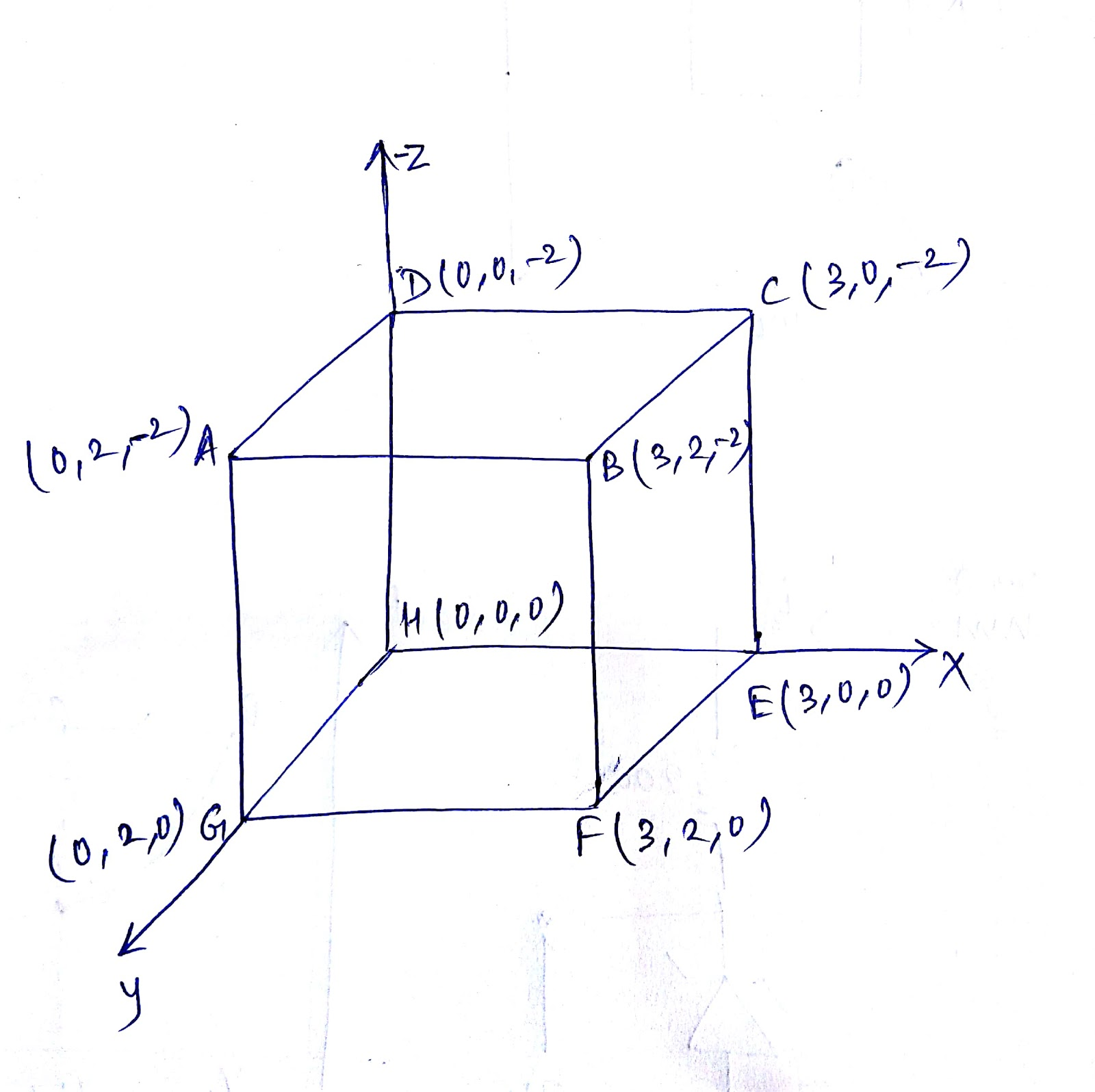Sol.

Flux via ABCD

Flux via BCEF

Stay tuned with us for further updates.